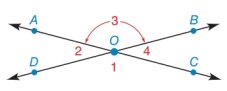Chapter 1.7, Problem 25E### Elementary Geometry for College St...

6th Edition
Daniel C. Alexander + 1 other
ISBN: 9781285195698

#### Solutions

Chapter
Section### Elementary Geometry for College St...

6th Edition
Daniel C. Alexander + 1 other
ISBN: 9781285195698
Textbook Problem
1 views

# In Exercises 19 to 26, use the drawing in which A C ↔ intersects D B ↔ at point O.If m ∠ 2 = x 2 − 10 and m ∠ 3 = x 3 + 40 , find x and m ∠ 2 .To determine

To find:

The value of x and m2.

Explanation

Given:

The given angles are m2=x210 and m3=x3+40.

The given figure is,

Definition:

The sum of measures of angles formed on straight line is supplementary angle.

Calculation:

From the given figure it can be observed that 2 and 3 are supplementary angles.

m2+m3=180°

Substitute x210 for m2 and x3+40 for m3 in above equation.

### Still sussing out bartleby?

Check out a sample textbook solution.

See a sample solution

#### The Solution to Your Study Problems

Bartleby provides explanations to thousands of textbook problems written by our experts, many with advanced degrees!

Get Started

#### In Exercises 63-68, use the graph of the function f to determine limxf(x) and limxf(x) 66.

Applied Calculus for the Managerial, Life, and Social Sciences: A Brief Approach

#### Solve the equations in Exercises 126. (x2+1)5(x+3)4+(x2+1)6(x+3)3=0

Finite Mathematics and Applied Calculus (MindTap Course List)

#### Solve sin2xsinx=0 if 0x2.

Trigonometry (MindTap Course List)

#### Find each value. logaa3

College Algebra (MindTap Course List)